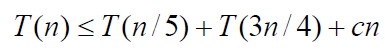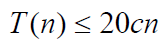# BFPRT算法：时间复杂度O(n)求第k小的数字（分治算法+快排）

step1 设置一个值r，将A分为长度为r的几个组。（假设r = 5）

A = { 0, 1, 2, 3, 4, 5, 6, 7, 8, 9, 10, 11, 12, 13, 14, 15, 16 };

G1               G2                   G3                  G4

step2 分别求取G1~4组别中的中值，G1 = 2, G2 = 7, G3 = 12, G4 = 15。

step3 求得G = {2, 7, 12, 15}数组中的中值，mid = 7。

//A[low..high]
int select_rank_k(int A[], int low, int high, int k)
{
int r_group = ceil((high - low + 1)*1.0 / r);//ceil取上限，总共分为r_group个组
//计算每个分组中值，存于A[]最前面
for (int i = 1; i <= r_group; ++i) {
sort(&A[low + (i - 1)*r], &A[(low + i*r - 1) > high ? high : (low + i*r - 1)]);
swap(A[low + i - 1], A[low + (i-1)*r + r / 2]);
}
//获得每个组的中值的中值(并置于A[low]位置，方便调用快排划分函数)
sort(&A[low], &A[low + r_group]);
swap(A[low], A[r_group / 2]);
int cur = partition(A, low, high);
if (cur == k-1){
return A[cur];
}
else if (cur < k){
return select_rank_k(A, cur + 1, high, k);
}
else{
return select_rank_k(A, low, cur - 1, k);
}
}

2018年6月4日修正

swap(A[low], A[r_group / 2]); 应该更改为swap(A[low], A[low+r_group / 2]);#include <stdio.h>
#include <algorithm>
#include <math.h>
using namespace std;

//划分——每次划分唯一确定一个元素位置
int partition(int A[], int low, int high)
{
int pivot = A[low];    //一般采用严蔚敏教材版本，以第1个位置为基准
while (low < high){
while (low < high && A[high] >= pivot){
--high;
}
A[low] = A[high];  //将比基准小的元素移动到左端
while (low < high && A[low] <= pivot){
++low;
}
A[high] = A[low];  //将比基准小的元素移动到右端
}
A[low] = pivot;
return low;
}

int r = 5;
//A[low..high]
int select_rank_k(int A[], int low, int high, int k)
{
int r_group = ceil((high - low + 1)*1.0 / r);//ceil取上限，总共分为r_group个组
//计算每个分组中值，存于A[]最前面
for (int i = 1; i <= r_group; ++i) {
sort(&A[low + (i - 1)*r], &A[(low + i*r - 1) > high ? high : (low + i*r - 1)]);
swap(A[low + i - 1], A[low + (i-1)*r + r / 2]);
}
//获得每个组的中值的中值(并置于A[low]位置，方便调用快排划分函数)
sort(&A[low], &A[low + r_group]);
swap(A[low], A[r_group / 2]);
int cur = partition(A, low, high);
if (cur == k-1){
return A[cur];
}
else if (cur < k){
return select_rank_k(A, cur + 1, high, k);
}
else{
return select_rank_k(A, low, cur - 1, k);
}
}

int main(void)
{
int A = { 0, 1, 2, 3, 4, 5, 6, 7, 8, 9, 10, 11, 12, 13, 14 };
printf("%d\n", select_rank_k(A, 0, 3, 2));
return 0;
}

@qingdujun

2017-11-22 北京 怀柔

Reference：陈玉福.计算机算法设计与分析，59-61

12-258017
03-21377707-311337
06-271万+
09-241万+
04-07176
11-15
10-14
04-111091
11-11149
12-291766
07-25358
08-16230
02-244689
09-14388
09-10543
07-16520
05-25600